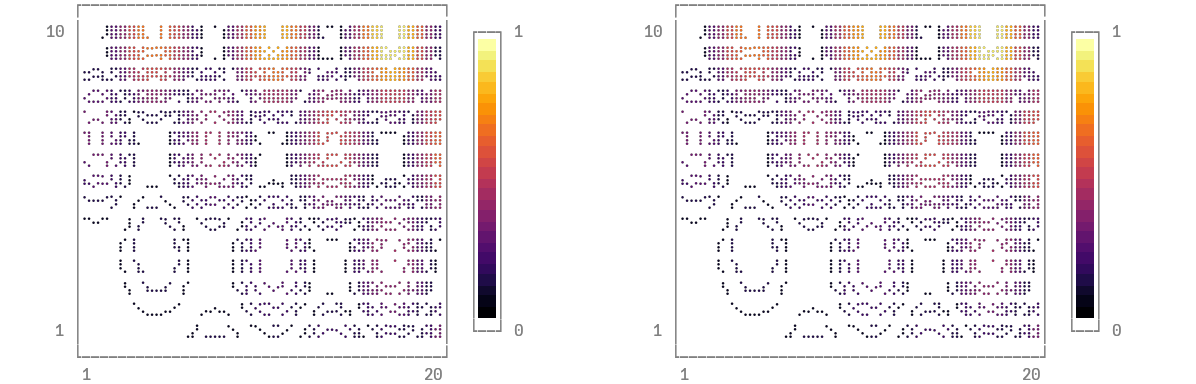# Contoursusing Plots
unicodeplots()

Any value for fill works here. We first build a filled contour from a function, then an unfilled contour from a matrix.

x = 1:0.5:20
y = 1:0.5:10
f(x, y) = begin
(3x + y ^ 2) * abs(sin(x) + cos(y))
end
X = repeat(reshape(x, 1, :), length(y), 1)
Y = repeat(y, 1, length(x))
Z = map(f, X, Y)
p1 = contour(x, y, f, fill = true)
p2 = contour(x, y, Z)
plot(p1, p2)